# Short Notes: Solid State – Part 2# Atomic Radius of an Atom in a Cubic System

Atomic Radius of an atom is half the distance between nearest neighbouring atom in a crystal. We express it in terms of length of the edge (a) of unit cell of the crystal.

(i) Simple cubic structure (sc) : Radius of atomas atoms touch each other along the edges.

(ii) Face centred cubic structure (fcc) : Radius of atomas the atoms touch each other along the face diagonal of the cube.

(iii) Body centred cubic structure (bcc) : Radius of atom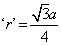as the atoms touch each other along the cross diagonal of the cube.

# Packing Efficiency

Packing efficiency is the percentage of total space filled by the particles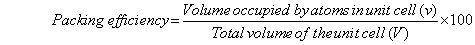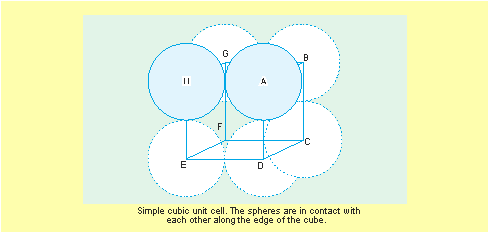#### (a) Packing efficiency in simple cubic structures:

Let ‘a’ be the cube edge and ‘r’ the atomic radius.

Thus, volume of the unit cell =a3

Since one atom is present in a unit cell, its volumeTherefore, 52% of unit cell is occupied by atoms and the rest 48% is empty space.

#### (b) Packing efficiency in ccp and hcp structures :

The efficiency of both types of packing, ccp and hcp, are equally efficient since in both atoms spheres occupy 74% of the available volume.

Now, we calculate the efficiency of packing in ccp structure.

Let the unit cell length be ‘a’ and face diagonal ‘b’ (see AC in Fig.). In this figure other sides are not shown for the sake of clarity.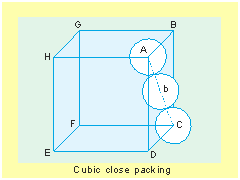Therefore, 74% of unit cell is occupied by atoms and the rest 26% to empty space.

#### (c) Packing efficiency in bcc structures :

In this case, the sphere (or atom) at the centre is in touch with other two atoms which are diagonally arranged (Fig.). Here, spheres along the body diagonal are shown with solid boundaries.,

The length of the body diagonal c is equal to r being the radius of the sphere. As all the three spheres along the diagonal touch each other.As already discussed, the total number of atoms associated in a bcc unit cell is 2, therefore, the volume (v) is,Therefore, 68% of unit cell is occupied by atoms and the rest 32% us empty space.

# Voids or Holes

In closed packed structures we see that the spheres (or atoms) are not 100% compactly packed due to their spherical shapes. Hence there must be some empty spaces when a layer of spheres are arranged; these empty spaces among the spheres are “Voids”.

The two dimensional arrangement of closed packing forms only triangular or trigonal voids between the three spheres whose apex may point to upward or downward.

In three dimensional arrangement of closed packing, two types of voids are possible tetrahedral voids and octahedral voids.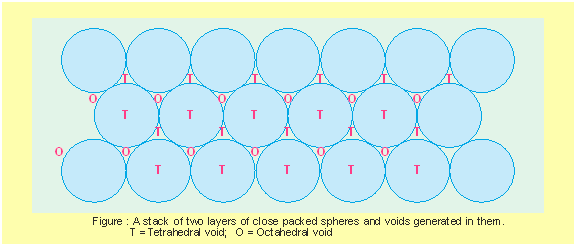#### (i) Tetrahedral voids :

When a sphere of second layer is above the trigonal void of first layer, it forms a tetrahedral void. This is because of the formation of a tetrahedral when the centres of these four spheres are joined.

There are two tetrahedral voids/per atom of crystal.

If R = radius of the spheres in closed packing.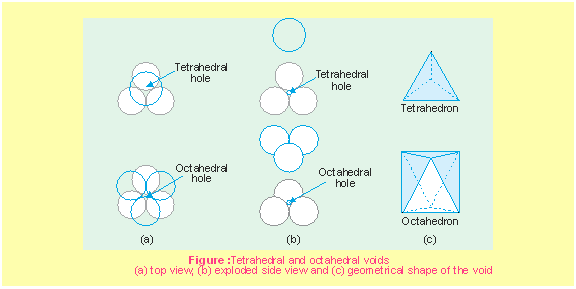#### (ii) Octahedral voids :

These types of voids are formed when the triangular voids in the second layer are above the triangular voids of first layer and the triangular shapes of these do not overlap. Such voids are surrounded by six spheres.

There are one octahedral void/per atom of crystal.In ionic compounds, generally anions being bigger, are present in the packing while the cations being smaller, occupy the voids.

(a) For cations occupyig tetrahedral voids

r+ = 0.225r

(b) For cations occupying the octahedral voids,

r+ = 0.414r

# Number of Voids filled and formula of a compound

We have already studied that when particles are closed packed (in ccp or hcp structures), two types of voids are generated, we find that

(i) Number of tetrahedral voids = 2 × Number of particles present in closed packing.

(ii) Number of octahedral voids = Number of particles present in closed packing.

In ionic compounds, if the cations are small in size, they may occupy tetrahedral voids, being smaller than octahedral voids. When cations are large enough to occupy the tetrahedral voids, they may occupy octahedral voids. Only a fraction of the total voids may be occupied.

So, knowing the fraction of the voids occupied, the formula of a given compound can be made or vice versa.

Example 1. A compound is formed by two elements A and B. Atoms of the element B (as anions) make ccp and those of the element A (as cations) occupy all the tetrahedral voids. What is the formula of the compound ?

Solution : As ccp lattice is formed by the element B, the number of tetrahedral voids generated

= 2 × number of atoms of B present in it.

Since, all the tetrahedral voids are occupied by the atoms of A, A = 2B

Thus, the atoms of elements A and B are present in the ratio = 1 : 2

Therefore, the formula of compound = AB2

Example 2. An element of body-centred cubic (bcc) structure has a cell edge of 288 pm. The density of the element is 7.2 g/cm3. How many atoms are present in 208 g of the element ?

Solution : Volume of 208g of the element# Locating Tetrahedral and Octahedral Voids

We know that closed packed structures have both tetrahedral and octahedral voids. In a ccp (fcc) structure, there are eight spheres at the corners of the unit cell and each sphere is in contact with three others giving rise to eight tetrahedral voids. Since ccp structure has 4 atoms/unit cell, the number of tetrahedral voids is twice the number of atoms.

In a ccp pattern, there is one octahedral void at the centre of body and 12 octahedral voids on each of the 12 edges of the cube. Each void on the edge is shared by four other unit cells.

Now, octahedral voids at the centre = 1Since, ccp structure has 4 atoms/unit cell, the number of octahedral voids is equal to the number of atoms.

# Imperfections in Crystal Solids

A solid crystal is composed of an aggregate of large number of small crystals. When crystallization process occurs at fast or moderate rate, single crystals are formed with defects. The defects are basically irregularities in the arrangement of constituent particles (atoms, ions or molecules).

Broadly two types of defects are found :

(i) Point Defects : When irregularities in the arrangement occurs around a point or an atom of the crystal.

(ii) Line Defects : When irregularities or deviations occur in the ideal arrangement along entire rows of the lattice points.

As per syllabus, we restrict our study to the point defects only.

# Point Defects

Point defects in non-ionic and ionic solids occur in different ways, as described by following chart:#### (i) Stoichiometric Defects :

Due to stoichiometric defects (also intrinsic or thermodynamic defects)  the stoichiometry (i.e., the ratio of cations and anions as per the chemical formula) of the solid is not disturbed.

Non-ionic solids show following defects:

##### (A) Vacancy Defects :

This defect arises due to a vacancy created in the lattice site. This results in decrease in density of the substance. Generally, this defect arises on heating of a substance.

##### (B) Interstitial Defect :

When some atoms or molecules occupy the interstitial site, this is interstitial defects. Due to this defect density of the substances increases.

Ionic solids have following defects instead of the above two defects because they must maintain electrical neutrality.

##### (C) Frenkel Defect :

Also called dislocation defect because in this defect cation (smaller ion) is dislocated from its normal site to an interstitial site. Thus vacancy defect arises at the original site while interstitial defect at the new location. As a result of this defect there is no change in the density of substance. This defect is shown by the ionic compounds having large difference in the size of ions. For example— ZnS, AgCl, AgBr, AgI, etc.

##### (D) Schottky Defect :

Basically it is a vacancy defect but in this case number of missing cations and anions are equal so that electrical neutrality of the substance is maintained. Like vacancy defect, Schottky defect also decreases the density of the substance. There is one Schottky defect per 1016 ions. This defect is shown by the ionic compounds having cations and anions of almost similar size. For example—NaCl, KCl, CsCl, AgBr, etc.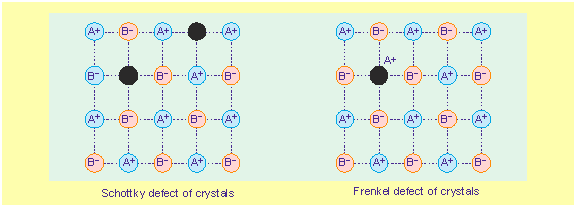#### (ii) Non-stoichiometric Defects :

Disturbance in the stoichiometry of a substance results into this type of defects. These defects may be of two types : (a) Metal excess defect and (b) Metal deficiency defect.

##### (A) Metal Excess Defect :

This defect may arise due to either anionic vacancies or presence of extra cations at interstitial sites.

(a) Due to anionic vacancies: Alkali halides like NaCl and KCl show this type of defect. For example, heating NaCl crystal in an atmosphere of Na-vapour deposits the excess of Na atoms on the surface of NaCl crystal. The Clions then diffuse to the surface of the crystal and combine with Na atoms to form NaCl. This happens by loss of electron by sodium atoms to form Na+ ions. The released electrons diffuse into the crystal and occupy anionic sites. As a result the crystal now has an excess of sodium.

F-Centres : These are the anionic sites occupied by the unpaired electrons. F-centres impart the colours to the crystals as a result of excitation of electrons when they absorb energy from the visible light falling on the crystals. For example—

Yellow colours of NaCl crystals due to excess of Na.

Pink colour of LiCl crystals due to excess of Li.

Violet colour of KCl crystals due to excess of K.

(b) Due to presence of extra cations of interstitial sites : This defect is like Frnekel defect, here also an extra cation occupies the interstitial site. In order to maintain electrical neutrality, an electron is present in another interstitial site.

For example, ZnO crystal (white) turns yellow when heated.##### (B) Metal Deficiency Defect :

This defect arises due to the missing of a cation from its lattice site and the presence of another cation having higher charge in the adjacent lattice site.

For example, the crystals of FeO is mostly found with a composition of FexO(x = 0.93 to 0.96), where some Fe2+ ions are missing and replaced by Fe3+ ion to maintain the total positive charge.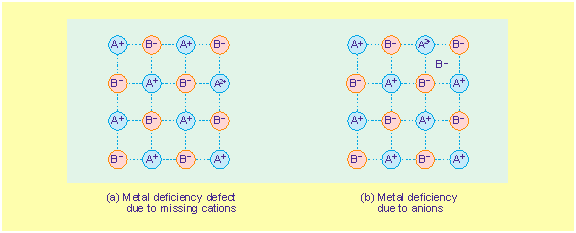(iii) Impurity Defect : Crystallization in some of the crystals, adds some foreign atoms. This is impurity defect.

For example, in melten NaCl, the sites of some Na+ ions are replaced by Sr2+ions and a little amount of SrCl2 is crystallized as impurity. Another similar examples are of CdCl2 and AgCl in solid solution.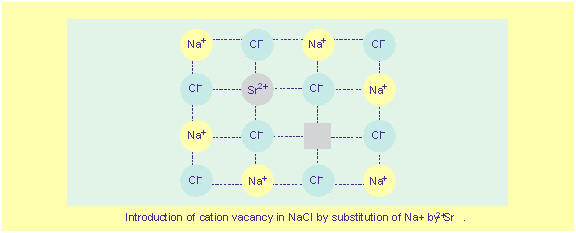# Electrical Properties of Solids

Solids are classified into three groups :

Conductors, Semiconductors and Insulators

#### (i) Conductors :

These generally include metals. Their conductivity is of the order of 107(ohmm)−1

#### (ii) Semiconductors :

Those solids which have intermediate conductivities ranging from 10−6 to 104(ohmm)−1 are classified as semiconductors. There is a rise in conductivity value as the temperature rises. Pure substances, like silicon and germanium, show conducting behaviour. The value of conductivity for these elements is too low at low temperatures. Doping is done to improve their conducting behaviour. As the temperature rises large number of valence electrons from the valence band jump to conduction band.

#### (iii) Insulators :

These are solids which have very low conductivity values ranging from 10−10 to 10−20(ohmm)−1. These are insulators.

#### (iv) Causes of Conductance in Solids :

In most of the solids and also in insulators conduction takes place due to migration of electrons under the influence of electric field. However, in ionic solids, it is the ions that are responsible for the conducting behaviour due to their movement.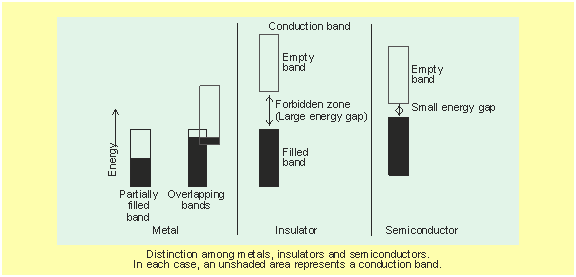The number of electrons available to participate in the conduction process determines the magnitude of electrical conductivity.

In metals, conductivity strongly depends upon the number of valence electrons available in an atom. Atomic orbitals form molecular orbitals and the closeness of these molecular orbitals forms a band.

If conduction band is not completely full or it lies very close to a higher unoccupied band, then electrons can jump from valence band to conduction band and some conductivity is observed (Fig.).

#### (v) Doping :

It is a process which introduces impurity in semiconductors to enhance its conductivity value.

#### (vi) n-type semiconductor :

When a silicon or germanium crystal is doped with group 15 element like P or As, the dopant atom forms four covalent bonds like a Si or Ge atom but the fifth electron, not used in bonding, becomes delocalised and contributes its share towards electrical conduction. Thus, silicon or germanium doped with P or As is n-type semiconductor (n stands for negative charge i.e. electron).

#### (vii) p-type semiconductor :

When silicon or germanium is doped with group 13 element like B or Al. The dopant is present only with three valence electrons. An electron vacancy or a hole is created at the place of missing fourth electron. Here, this hole moves through the crystal like a positive charge giving rise to electrical conductivity. Thus Si or Ge doped with B or Al is p-type of semi conductor, (p stands for positive hole) since it is the positive hole that is responsible for conduction.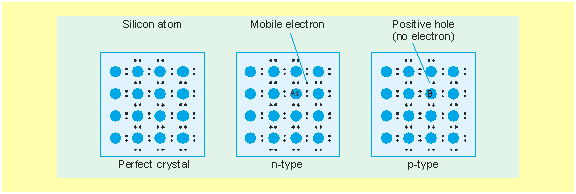#### (ix) Diode :

Combining n-type and p-type conductors results in a diode. These diodes are used as rectifiers.

#### (x) Transistors :

These detect or amplify radio or audio signals. They consist of pnp or npn type of semiconductors.

#### (xi) Photo-diode :

These are diodes which are capable of converting light energy into electrical energy and are put to use in solar cells.

# Magnetic Properties of Solids

Solids can be classified on the basis of their magnetic properties.

#### (a) Diamagnetic :

Weakly repelled by the external magnetic field. This substance has fully-filled orbitals. Magnetic moment of an electron with spin in one direction is cancels the magnetic moment of other electron with spin in opposite direction. Examples:TiO2, V2O3,  NaCl, benzene, etc.

#### (b) Paramagnetic :

(i) Weakly attracted by the external magnetic field. (ii) Paramagnetism is a temporary effect and the substance loses its magnetism in the absence of external magnetic field.

Atoms, ions or molecules of this type of substance contain unpaired electrons. Examples : TiO, VO2, CuO, O2, Cu2+, Fe3+, etc.#### (c) Ferromagnetic :

(i) Strongly attracted by the external field. (ii) Exhibits magnetism, even in the absence of magnetic field. (iii) These substances can be permanently magnetized.

It arises due to spontaneous alignment of magnetic moments in the same direction.

Example : Fe, Ni, Co, CrO2.

#### (d) Antiferromagnetic :

Zero net magnetic moment, but expected to possess paramagnetism because of the presence of unpaired electrons.

The resulting magnetic moment is zero, because of the presence of equal number of magnetic moments in opposite direction.

Examples : MnO, Mn2O2, MnO4, V2O3, Fe2O3, FeO, CoO, Co3O4, NiO

#### (e) Ferrimagnetic :

Small net magnetic moment, but expected to possess large magnetism on the basis of the unpaired electrons.

Due to the unequal number of magnetic moments of opposite direction, the net or resulting magnetic moment is small. Examples : Fe3O4

#### (f) Magnetic Moments :

The magnetic properties of substances result from their magnetic moments associated with individual electron. Each electron has a magnetic moment, origin of which lies in two sources i.e., rotation of electron (spins) around its own axis and simultaneously revolution around the nucleus (orbital motion).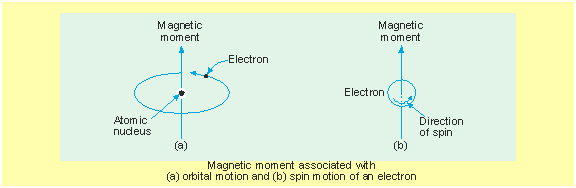An electron in motion is just like a small current loop. Two types of motions give rise to two types of magnetic moments, spin and orbital magnetic moments (Fig.). Spin moment is directed along the spin axis and is in up or down direction (Fig.). Orbital motion also generates a magnetic field and thus give rise to orbital moment along its axis of rotation (Fig.). In this way each electron of the atom behaves like a small bar magnet having permanent orbital and spin magnetic moments. We measure magnetic moments in Bohr magneton unit (B. M. unit)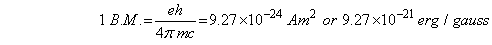where e is charge on electron; h is Planck’s constant; m is the mass of electron and c is the velocity of light.

Depending upon two spin motions (clockwise and anticlockwise), spin magnetic moment may aquire two values ±μB. Contribution of the orbital magnetic moment is equal to mlB, where mlis magnetic quantum number of electron.

#### (g) Curie-Temperature :

The temperature at which a ferromagenetic substance loses its ferromagnetism and attains paramagnetism is Curie-temperature. For iron the curie-temperature is 1033 K, for Ni it is 629 K and for Fe3O4 it is 850 K. Below this tempperature paramagnetics behave as ferromagnetics.

# Crystal Behaviour of Some Ionic Solids

#### (a) NaCl (fcc) :

Cl in ccp, Na+ ions occupy all the octahedral voids.

Coordination number 6 : 6 and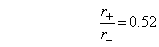Number of formula units per unit cell = 4

#### (b) CsCl (bcc) :

Cl in cubic arrangement Cs+ ions occupy cubic voids.

Coordination number 8 : 8 andNumber of formula units per unit cell = 1

#### (c) ZnS (Zinc blende) :

S2− form fcc lattice and Zn2+  ions in alternate tetrahedral voids, coordination number 4 : 4 and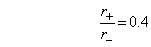Number of formula units per unit cell = 4

#### (d) ZnS (Wurtzite) :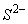form hcp structure and Zn2+ ions in alternate tetrahedral voids, coordination number 4 : 4 andNumber of formula units per unit cell = 4

#### (e) CaF2 (Fluorite) :

Ca2+ ions in ccp and F ions occupy all tetrahedral voids, coordination number 8 : 4 and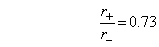Number of formula units per unit cell = 4

#### (f) Na2O (Antifluorite structure) :

O2− ions in ccp and Na+ions occupy all tetrahedral voids, coordination number 4 : 8.